Do you like the article?
Share it with others -

Use new possibilities of MetaTrader 5

# Applying Monte Carlo method in reinforcement learning

26 February 2019, 15:52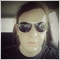15
13 437

### Brief summary of the previous article and the algorithm improvement methods

In the previous article, we considered the Random Decision Forest algorithm and wrote a simple self-learning EA based on Reinforcement learning.

The following main advantage of this approach was outlined:

• ease of developing a trading algorithm and high "learning" speed. Reinforcement learning (RL) is easily incorporated into any trading EA and speeds up its optimization.

At the same time, this approach has one major drawback:

• the algorithm is prone to over-optimization (overfitting). In other words, it is characterized by weak generalization on the general population with unknown outcomes distribution. This means that it does not look for real fundamental market patterns characteristic of the entire historical period of a financial instrument, but rather over-fits based on the current market situation, while the global patterns remain on the other side of the trained agent's “understanding”. However, genetic optimization has the same drawback and works much slower in case of a large number of variables.

There are two main methods to combat overfitting:

• Feature engineering, or constructing attributes. The main objective of this approach is the selection of such features and a target variable that would describe the general population with a low error. In other words, the objective is to search for plausible regularities by statistical and econometric methods using predictors enumeration. In non-stationary markets, this task is rather complicated and, for certain strategies, insoluble. However, we should strive to choose the optimal strategy.
• Regularization is used to roughen the model by making amends at the level of the applied algorithm. From the previous article, we remember that the r parameter is used for that in RDF. Regularization allows achieving the balance of errors between the training and the test samples increasing the stability of the model on new data (when it is possible).

### Improved approach to Reinforcement learning

The above techniques are included in the algorithm in the original way. On the one hand, constructing the attributes is performed via enumerating price increments and selecting several best ones, while on the other hand, the models with the smallest classification error on new (out-of-bag) data are selected via tuning the r parameter.

In addition, there is a new opportunity to simultaneously create several RL agents you can set different settings to, which, in theory, should increase the stability of the model on the new data. The models enumeration is performed in the optimizer using the Monte Carlo method (random sampling of labels), while the best model is written to a file for further use.

### Creating the CRLAgent base class

For convenience, the library is based on OOP, which makes it easy to connect it to an EA and declare a required number of RL agents.

Here I will describe some class fields for a deeper understanding of the interaction structure within the program.

```//+------------------------------------------------------------------+
//|RL agent base class                                               |
//+------------------------------------------------------------------+
class CRLAgent
{
public:
CRLAgent(string,int,int,int,double, double);
~CRLAgent(void);
static int        agentIDs;

void              updatePolicy(double,double&[]); //Update the learner policy after each deal
void              updateReward();                 //Update reward after closing a deal
int               trees;
double            r;
int               features;
double            rferrors[], lastrferrors[];
string            Name;
```

The first three methods are used to form the policy (strategy) of the learner (agent), update the rewards and receive a trading signal from a trained agent. They are described in detail in the first article.

Auxiliary fields defining the random forest settings, number of attributes (inputs), arrays for storing model errors and the agent (agents group) name are declared further on.

```private:
CMatrixDouble     RDFpolicyMatrix;
CDecisionForest   RDF;
CDFReport         RDF_report;

double            RFout[];
int               RDFinfo;
int               agentID;
int               numberOfsamples;
void              getRDFstructure();
double            getLastProfit();
int               getLastOrderType();
void              RecursiveElimination();
double            bestFeatures[];
int               bestfeatures_num;
double            prob_shift;
bool random;
};```

The matrix for saving the parametrized learner policy, random forest object and auxiliary object for storing errors are declared further on.

The following static variable is provided for storing an agent's unique ID:

`static int CRLAgent::agentIDs=0;`

The constructor initializes all variables before starting the work:

```CRLAgent::CRLAgent(string AgentName,int number_of_features, int bestFeatures_number, int number_of_trees,double regularization, double shift_probability) {
random=false;
MathSrand(GetTickCount());
ArrayResize(rferrors,2);
ArrayResize(lastrferrors,2);
Name = AgentName;
ArrayResize(RFout,2);
trees = number_of_trees;
r = regularization;
features = number_of_features;
bestfeatures_num = bestFeatures_number;
prob_shift = shift_probability;
if(bestfeatures_num>features) bestfeatures_num = features;
ArrayResize(bestFeatures,1);
numberOfsamples = 0;
agentIDs++;
agentID = agentIDs;
getRDFstructure();
}```

At the very end, the control is delegated to the getRDFstructure() method, which performs the following actions:

```//+------------------------------------------------------------------+
//+------------------------------------------------------------------+
CRLAgent::getRDFstructure(void) {
string path=_Symbol+(string)_Period+Name+"\\";
if(MQLInfoInteger(MQL_OPTIMIZATION)) {
if(FileIsExist(path+"RFlasterrors"+(string)agentID+".rl",FILE_COMMON)) {
int getRDF;
do {
FileClose(getRDF);
}
while (getRDF<0);
}
else {
int getRDF;
do {
getRDF=FileOpen(path+"RFlasterrors"+(string)agentID+".rl",FILE_WRITE|FILE_BIN|FILE_ANSI|FILE_COMMON);
double arr;
ArrayInitialize(arr,1);
FileWriteArray(getRDF,arr,0);
FileClose(getRDF);
}
while (getRDF<0);
}
return;
}

if(FileIsExist(path+"RFmodel"+(string)agentID+".rl",FILE_COMMON)) {
CSerializer serialize;
string RDFmodel="";
while(FileIsEnding(getRDF)==false)

FileClose(getRDF);
serialize.UStart_Str(RDFmodel);
CDForest::DFUnserialize(serialize,RDF);
serialize.Stop();

FileClose(getRDF);

FileClose(getRDF);

getRDF=FileOpen(path+"RFlasterrors"+(string)agentID+".rl",FILE_WRITE|FILE_BIN|FILE_ANSI|FILE_COMMON);
double arr;
ArrayInitialize(arr,1);
FileWriteArray(getRDF,arr,0);
FileClose(getRDF);
}
else random = true;
}```

If the EA optimization is started, the files are checked for the errors recorded during the previous optimizer iterations. Model errors are compared at each new iteration for the subsequent selection of the smallest one.

If the EA is launched in the test mode, the trained model is downloaded from the files for further use. Also, the model's latest errors are erased and default values equal to one are set so that the new optimization starts from zero.

After the next run in the optimizer, the learner is trained as follows:

```//+------------------------------------------------------------------+
//|Learn an agent                                                    |
//+------------------------------------------------------------------+
double CRLAgent::learnAnAgent(void)
{
if(MQLInfoInteger(MQL_OPTIMIZATION)) {
if(numberOfsamples>0) {
RecursiveElimination();```

Control is delegated to the specified method intended for sequential selection of attributes, namely price increments. Let's see how it works:

```//+------------------------------------------------------------------+
//|Recursive feature elimitation for matrix inputs                   |
//+------------------------------------------------------------------+
CRLAgent::RecursiveElimination(void) {
//feature transformation, making every 2 features as returns with different lag's
ArrayResize(bestFeatures,0);
ArrayInitialize(bestFeatures,0);
CDecisionForest   mRDF;
CMatrixDouble     m;
CDFReport         mRep;
m.Resize(RDFpolicyMatrix.Size(),3);
int modelCounterInitial = 0;

for(int bf=1;bf<features;bf++) {
for(int i=0;i<RDFpolicyMatrix.Size();i++) {
m[i].Set(0,RDFpolicyMatrix[i]/RDFpolicyMatrix[i][bf]);	                                //fill the matrix with increments (array zero index price is divided by the price with the bf shift)
m[i].Set(1,RDFpolicyMatrix[i][features]);
m[i].Set(2,RDFpolicyMatrix[i][features+1]);
}
CDForest::DFBuildRandomDecisionForest(m,RDFpolicyMatrix.Size(),1,2,trees,r,RDFinfo,mRDF,mRep);	//Train a random forest where only a selected increment is used as a predictor
ArrayResize(bestFeatures,ArrayRange(bestFeatures,0)+1);
bestFeatures[modelCounterInitial] = mRep.m_oobrelclserror;	                                //save the error on the oob set
bestFeatures[modelCounterInitial] = bf;	                                                //save the increment "lag"
modelCounterInitial++;
}

ArraySort(bestFeatures);                                                                              //sort the array (by zero dimension), i.e. oob appears by error here
ArrayResize(bestFeatures,bestfeatures_num);                                                           //leave only the best bestfeatures_num attributes

m.Resize(RDFpolicyMatrix.Size(),2+ArrayRange(bestFeatures,0));

for(int i=0;i<RDFpolicyMatrix.Size();i++) {                                                           // fill the matrix again, but this time, with the best attributes
for(int l=0;l<ArrayRange(bestFeatures,0);l++)
{
m[i].Set(l,RDFpolicyMatrix[i]/RDFpolicyMatrix[i][(int)bestFeatures[l]]);
}
m[i].Set(ArrayRange(bestFeatures,0),RDFpolicyMatrix[i][features]);
m[i].Set(ArrayRange(bestFeatures,0)+1,RDFpolicyMatrix[i][features+1]);
}

CDForest::DFBuildRandomDecisionForest(m,RDFpolicyMatrix.Size(),ArrayRange(bestFeatures,0),2,trees,r,RDFinfo,RDF,RDF_report);  // train a random forest on the selected best attributes
}```

Let's have a look at the agent training method in its entirety:

```//+------------------------------------------------------------------+
//|Learn an agent                                                    |
//+------------------------------------------------------------------+
double CRLAgent::learnAnAgent(void)
{
if(MQLInfoInteger(MQL_OPTIMIZATION)) {
if(numberOfsamples>0) {
RecursiveElimination();
if(RDF_report.m_oobrelclserror<lastrferrors) {
string path=_Symbol+(string)_Period+Name+"\\";
//FileDelete(path+"RFmodel"+(string)agentID+".rl",FILE_COMMON);

CSerializer serialize;
serialize.Alloc_Start();
CDForest::DFAlloc(serialize,RDF);
serialize.SStart_Str();
CDForest::DFSerialize(serialize,RDF);
serialize.Stop();

int setRDF;

do {
setRDF=FileOpen(path+"RFlasterrors"+(string)agentID+".rl",FILE_WRITE|FILE_BIN|FILE_ANSI|FILE_COMMON);
if(setRDF<0) continue;
lastrferrors=RDF_report.m_relclserror;
lastrferrors=RDF_report.m_oobrelclserror;
FileWriteArray(setRDF,lastrferrors,0);
FileClose(setRDF);

setRDF=FileOpen(path+"RFmodel"+(string)agentID+".rl",FILE_WRITE|FILE_TXT|FILE_COMMON);
FileWrite(setRDF,serialize.Get_String());
FileClose(setRDF);

setRDF=FileOpen(path+"RFerrors"+(string)agentID+".rl",FILE_WRITE|FILE_BIN|FILE_ANSI|FILE_COMMON);
rferrors=RDF_report.m_relclserror;
rferrors=RDF_report.m_oobrelclserror;
FileWriteArray(setRDF,rferrors,0);
FileClose(setRDF);

setRDF=FileOpen(path+"Kernel"+(string)agentID+".rl",FILE_WRITE|FILE_BIN|FILE_ANSI|FILE_COMMON);
FileWriteArray(setRDF,bestFeatures);
FileClose(setRDF);
}
while(setRDF<0);
}
}
}
return 1-RDF_report.m_oobrelclserror;
}```

After selecting the attributes and training the agent, the agent classification error on the current optimization pass is compared with the minimum error saved during the entire optimization. If the current agent's error is less, then the current model is saved as the best one, and subsequent comparisons use this model's error.

The Monte Carlo method (random sampling of price variables) should be considered separately:

```//+------------------------------------------------------------------+
//+------------------------------------------------------------------+
double res=0.5;
if(!MQLInfoInteger(MQL_OPTIMIZATION) && !random) {
double kerfeatures[];
ArrayResize(kerfeatures,ArrayRange(bestFeatures,0));
ArrayInitialize(kerfeatures,0);

for(int i=0;i<ArraySize(kerfeatures);i++) {
kerfeatures[i] = featuresValues/featuresValues[(int)bestFeatures[i]];
}

CDForest::DFProcess(RDF,kerfeatures,RFout);
return RFout;
}
else {
if(countOrders()==0) if(rand()/32767.0<0.5) res = 0; else res = 1;
else {
if(countOrders(0)!=0) if(rand()/32767.0>prob_shift) res = 0; else res = 1;
if(countOrders(1)!=0) if(rand()/32767.0<prob_shift) res = 0; else res = 1;
}
}
return res;
}```

If the EA is not in the optimization mode, the already trained model downloaded when initializing the EA is used to receive trading signals. Otherwise, if the optimization process is in progress or if there are no model files, the signals occur randomly in the absence of open positions (50/50) and with an offset probability set by the prob_shift variable in the presence of open orders. Thus, for example, if an open buy transaction already exists, you can shift the probability of a sell signal to 0.1 (instead of 0.5). As a result, the total number of samples in the training set decreases and positions are held longer. At the same time, when setting prob_shift >= 0.5, the number of deals increases.

### Creating the CRLAgents class

Now we can have multiple agents (learners) performing various tasks in a trading system. The present class has been developed for the more convenient management of the homogeneous learner groups.

```//+------------------------------------------------------------------+
//|Multiple RL agents class                                          |
//+------------------------------------------------------------------+
class CRLAgents
{
private:
struct Agents
{
double            inpVector[];
CRLAgent         *ag;
double            rms;
double            oob;
};

void              getStatistics();
string            groupName;
public:
CRLAgents(string,int,int,int,int,double,double);
~CRLAgents(void);
Agents            agent[];
void              updatePolicies(double);
void              updateRewards();
double            learnAllAgents();
void              setAgentSettings(int,int,int,double);
};```

The Agents structure accepts the parameters of each learner, and the array of structures contains their total number. In case of a single agent, it also makes sense to use this particular class.

The constructor takes all the necessary parameters for learning:

```CRLAgents::CRLAgents(string AgentsName,int agentsQuantity,int features, int bestfeatures, int treesNumber,double regularization, double shift_probability)
{
groupName=AgentsName;
ArrayResize(agent,agentsQuantity);
for(int i=0;i<agentsQuantity;i++) {
ArrayResize(agent[i].inpVector,features);
ArrayInitialize(agent[i].inpVector,0);
agent[i].ag  = new CRLAgent(AgentsName, features, bestfeatures, treesNumber, regularization, shift_probability);
agent[i].rms = agent[i].ag.rferrors;
agent[i].oob = agent[i].ag.rferrors;
}
}```

They include: agents group name, number of workers, number of attributes for each worker, number of the best selected attributes, number of trees in the forest, regularization parameter (separation into training and test sets) and offset of the probability to manage the number of trades.

As we can see, the learner objects with the same inputs, as well as training and test errors are placed to the structure array.

The agents learning method calls the learning method for each of the CRLAgent base class and returns the average error on a test set for all agents:

```//+------------------------------------------------------------------+
//|Learn all agents                                                  |
//+------------------------------------------------------------------+
double CRLAgents::learnAllAgents(void){
double err=0;
for(int i=0;i<ArraySize(agent);i++)
err+=agent[i].ag.learnAnAgent();
return err/ArraySize(agent);
}```

This error is used as a custom optimization criteria to visualize the error spread when iterating models using the Monte Carlo method.

When creating a certain number of learners in one subgroup, their settings remain the same. Therefore, there is a method for adjusting the parameters of each individual learner:

```//+------------------------------------------------------------------+
//|Change agents settings                                            |
//+------------------------------------------------------------------+
CRLAgents::setAgentSettings(int agentNumber,int features,int bestfeatures,int treesNumber,double regularization,double shift_probability) {
agent[agentNumber].ag.features=features;
agent[agentNumber].ag.bestfeatures_num=bestfeatures;
agent[agentNumber].ag.trees=treesNumber;
agent[agentNumber].ag.r=regularization;
agent[agentNumber].ag.prob_shift=shift_probability;
ArrayResize(agent[agentNumber].inpVector,features);
ArrayInitialize(agent[agentNumber].inpVector,0);
}```

Unlike the CRLAgent base class, in CRLAgents, a trading signal is displayed as the average for signals of all learners belonging to a subgroup:

```//+------------------------------------------------------------------+
//+------------------------------------------------------------------+
double signal[];
double sig=0;
ArrayResize(signal,ArraySize(agent));

for(int i=0;i<ArraySize(agent);i++)
return sig/(double)ArraySize(agent);
}```

Finally, the method of obtaining statistics displays data on errors concerning test and training of all agents at the end of a single pass in the tester:

```//|Get agents statistics                                             |
//+------------------------------------------------------------------+
void CRLAgents::getStatistics(void)
{
double arr[];
double arrrms[];
ArrayResize(arr,ArraySize(agent));
ArrayResize(arrrms,ArraySize(agent));

for(int i=0;i<ArraySize(agent);i++) {
arrrms[i]=agent[i].rms;
arr[i]=agent[i].oob;
}

Print(groupName+" TRAIN LOSS");
ArrayPrint(arrrms);
Print(groupName+" OOB LOSS");
ArrayPrint(arr);
}```

### Developing a trading robot based on RL Monte Carlo library

Now we only have to write a simple EA to demonstrate the library features. Let's start from the first case, in which only one agent is created to be trained on trading instrument's Close prices.

`#include <RL Monte Carlo.mqh>`
```input int       number_of_passes = 10;
input double    shift_probab = 0,5;
input double    regularize=0.6;
sinput int      number_of_best_features = 5;
sinput double   treshhold = 0.5;
sinput double   MaximumRisk=0.01;
sinput double   CustomLot=0;

CRLAgents *ag1=new CRLAgents("RlMonteCarlo",1,500,number_of_best_features,50,regularize,shift_probab);```

Include the library and define inputs to be optimized. number_of_passes is meant for determining the number of passes in the terminal optimizer and is not passed anywhere. Since entries and exits are randomly selected by the worker, it is possible to achieve an optimal strategy after multiple passes and defining the smallest error. The more passes installed, the higher the probability of obtaining the optimal strategy.

The remaining settings are outlined above and passed directly to the created model. Here, we have created a single agent belonging to the RlMonteCarlo group. 500 attributes are passed to its input and 5 best ones are to be selected among them. The model is to have 50 decision trees with a separation of training and test sets 0.6 (r parameter) without the probability offset.

In the OnTester function, the custom optimization criterion (as an averaged error on a test sample along all the learners) is returned after preliminarily training them:

```//+------------------------------------------------------------------+
//| Expert ontester function                                         |
//+------------------------------------------------------------------+
double OnTester() {
if(MQLInfoInteger(MQL_OPTIMIZATION)) return ag1.learnAllAgents();
else return NULL;
}```

Learners are deleted and the memory is released during deinitialization:

```//+------------------------------------------------------------------+
//| Expert deinitialization function                                 |
//+------------------------------------------------------------------+
void OnDeinit(const int reason) {
delete ag1;
}```

The predictors' vector is filled as follows:

```//+------------------------------------------------------------------+
//| Calculate Tsignal                                                |
//+------------------------------------------------------------------+
void calcTsignal() {
Tsignal=0;
for(int i=0;i<ArraySize(ag1.agent);i++) {
CopyClose(_Symbol,0,1,ArraySize(ag1.agent[i].inpVector),ag1.agent[i].inpVector);
ArraySetAsSeries(ag1.agent[i].inpVector,true);
}
}```

The last 500 Close prices are simply taken in this case. As you may remember, the ratio of the array zero element to some other one (with a certain lag) is considered a predictor in a model, therefore, set the array accepting Close prices, as series. The trading signal obtaining method is called after that.

The last function is a trading one:

```//+------------------------------------------------------------------+
//| Place orders                                                     |
//+------------------------------------------------------------------+
void placeOrders() {
for(int b=OrdersTotal()-1; b>=0; b--)
if(OrderSelect(b,SELECT_BY_POS)==true) {
if(OrderType()==0 && Tsignal>0.5) if(OrderClose(OrderTicket(),OrderLots(),OrderClosePrice(),0,Red)) {ag1.updateRewards();}
if(OrderType()==1 && Tsignal<0.5) if(OrderClose(OrderTicket(),OrderLots(),OrderClosePrice(),0,Red)) {ag1.updateRewards();}
}

if(countOrders(0)!=0 || countOrders(1)!=0) return;
if(Tsignal>0.5+treshhold && (OrderSend(Symbol(),OP_SELL,lotsOptimized(),SymbolInfoDouble(_Symbol,SYMBOL_BID),0,0,0,NULL,OrderMagic,INT_MIN)>0)) { ag1.updatePolicies(Tsignal); }
}```

The 'threshold' parameter has been additionally introduced. It allows setting the signal activation threshold. For example, if the buy signal probability is less than 0.6, the order is not opened.

### Optimizing the RL Monte Carlo Trader EA

Let's have a look at the settings that can be optimized: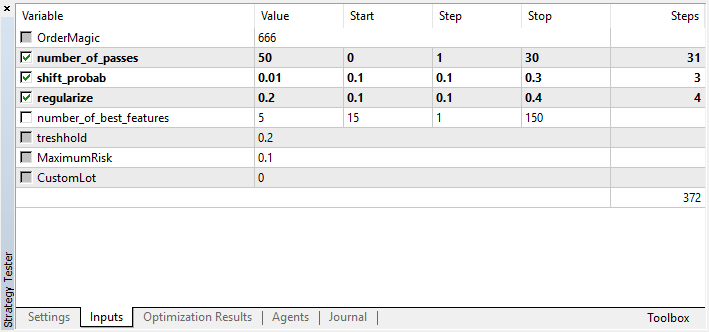Keep in mind that number_of_passes does not pass any values to the learner. Instead, it simply sets the number of optimizer passes. Suppose that you have made your mind about other settings, and now you want to use exclusively Monte Carlo-based enumeration. In that case, you should optimize only by this criterion. You can still optimize the remaining four settings if you want.

Another feature of the current version is that there is no need to disable test agents, since the passes in the optimizer are independent of each other and the sequence of saving the models is not important.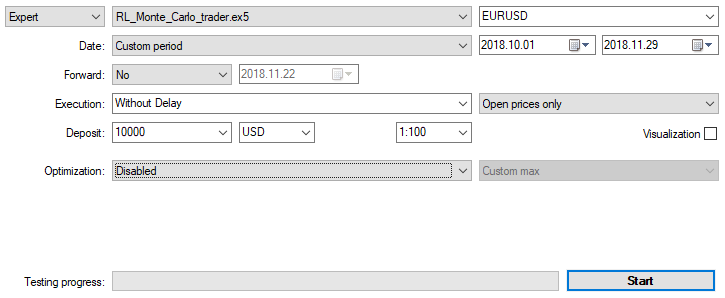Let's optimize the EA with the settings specified above on M15 by Open prices within two months. "Custom max" should be selected as an optimization criterion. Optimization process can be stopped at any moment when an acceptable value of the optimization criterion is reached: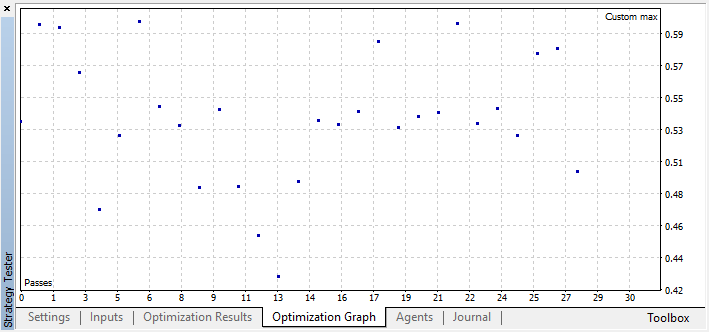For example, I stopped the optimization at step 44, because one of the best models exceeded the accuracy threshold of 0.6. This means that the classification error on the test sample fell below 0.4. Keep in mind that the better the model, the lower the error, but for the correct operation of the genetic algorithm (if you wish to use it), the error values are inverted.

You can check the best model settings in the Optimization tab sorting the values by custom criterion maximum: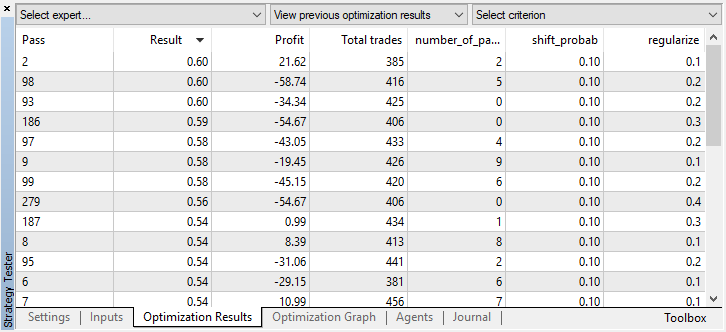In this case, the best model is obtained with a probability offset of 0.1 and with the r parameter of 0.2 (the training set is only 20% of the entire deals matrix, while 80% is a test subset).

After stopping the optimization, simply enable the single test mode (since the best model is written to the file and only that model is to be uploaded):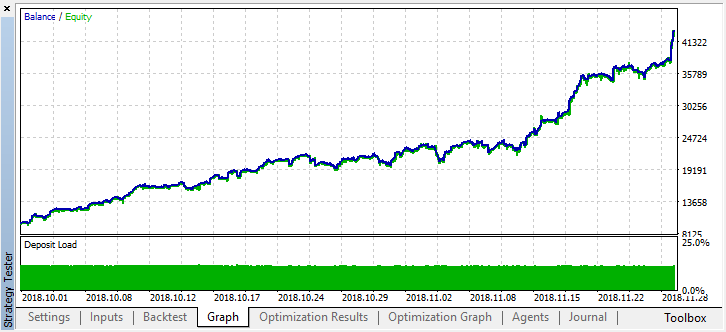Let's scroll the history for two months back and see how the model works for the full four months: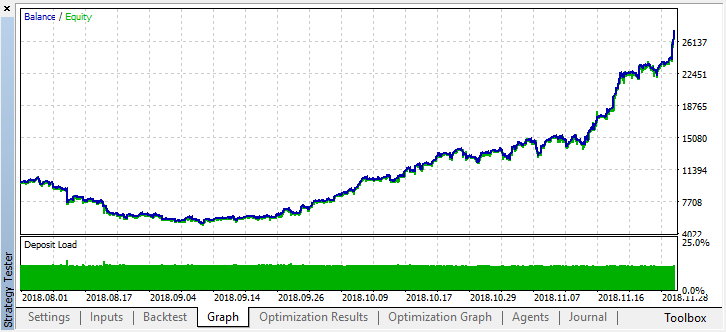We can see that the resulting model lasted another month (almost the entire September), while breaking down in August. Let's try to fine-tune the model by setting 'treshhold' to 0.2: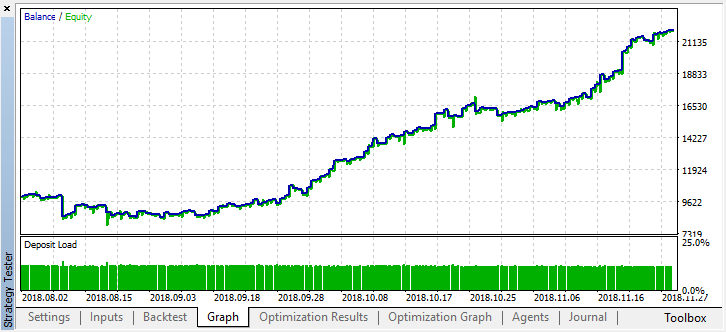It has become noticeably better. The model's accuracy has increased, while the number of deals has been reduced. Testing for greater depth is possible provided that the training period has had the appropriate length.

Now let's consider the EA variant featuring several learners in order to compare efficiency of the multi-agent approach with that of the single-agent one.

To do this, add the "Multi" ending when creating a group of agents, so that the files of different systems are not mixed. Also, set the number of workers, for example, five:

`CRLAgents *ag1=new CRLAgents("RlMonteCarloMulti",5,500,number_of_best_features,50,regularize,shift_probab);`

All the agents have turned out to be the same (they have identical settings). You can configure each worker separately in the EA initialization function:

```//+------------------------------------------------------------------+
//| Expert initialization function                                   |
//+------------------------------------------------------------------+
int OnInit() {
ag1.setAgentSettings(0,500,20,50,regularize,shift_probab);
ag1.setAgentSettings(1,200,15,50,regularize,shift_probab);
ag1.setAgentSettings(2,100,10,50,regularize,shift_probab);
ag1.setAgentSettings(3,50,5,50,regularize,shift_probab);
ag1.setAgentSettings(4,25,2,50,regularize,shift_probab);
return(INIT_SUCCEEDED);
}```

Here I decided not to complicate things any further and located the number of attributes for agents in descending order from 500 to 25. Also, the number of the best selected attributes was decreased from 20 to two. Other settings were left unchanged. You can change them and add new optimized parameters. I encourage you to experiment with the settings and share your results in the comments below.

As you may remember, the arrays are filled with predictor values in the function:

```//+------------------------------------------------------------------+
//| Calculate Tsignal                                                |
//+------------------------------------------------------------------+
void calcTsignal() {
Tsignal=0;
for(int i=0;i<ArraySize(ag1.agent);i++) {
CopyClose(_Symbol,0,1,ArraySize(ag1.agent[i].inpVector),ag1.agent[i].inpVector);
ArraySetAsSeries(ag1.agent[i].inpVector,true);
}
}```

Here we simply fill the inpVector array with Close prices for each learner depending on its size, therefore the function is universal for this case and does not need to be changed.

Launch the optimization with the same settings as for a single agent: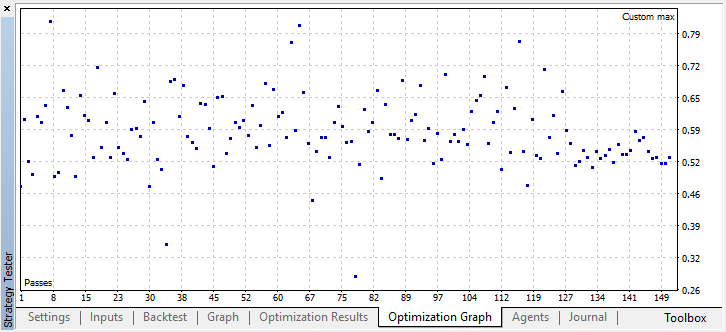The best result exceeded 0.7, which is much better than in the first case. Launch a single run in the tester: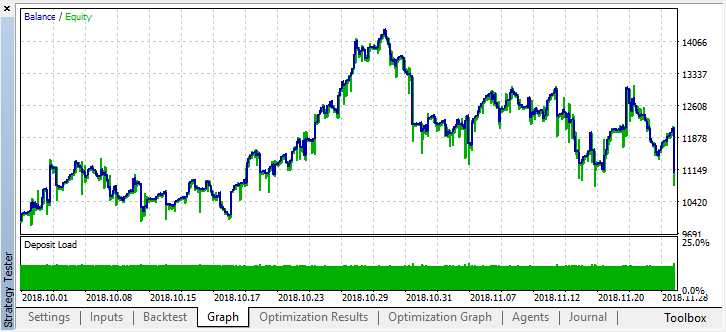The real result reflected by the balance graph became much worse. Why? Let's look at the number of random trades of the best run. There are only 21 of them!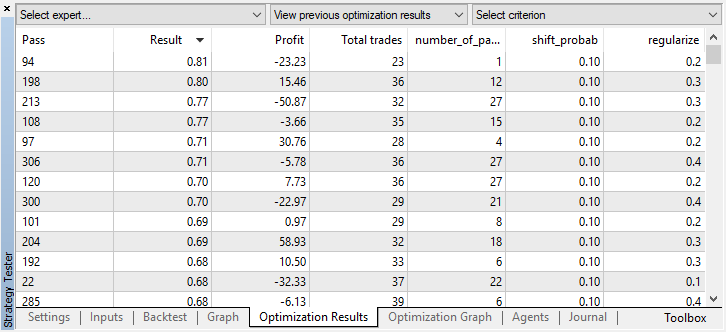It turned out this way because the signals of several agents overlapped due to random sampling, and the total number of trades decreased. To fix this, set the shift_probab parameter closer to 0.5. In this case, the number of deals for each individual agent will be greater, thus increasing the number of total transactions as well. On the other hand, you can simply increase the learning period, but first we should see whether it is possible to work with such a model further. Set 'threshold' to 0.2 and see what happens: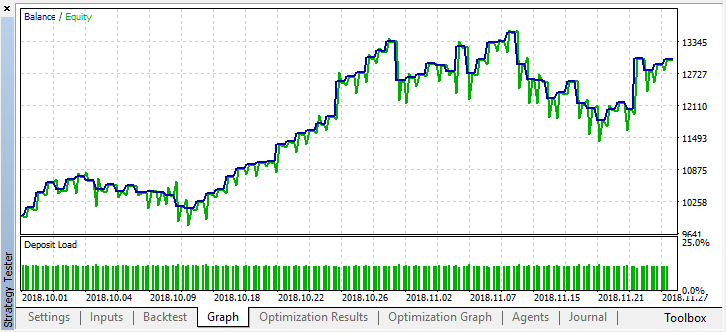At least, the model does not lose money, although the number of deals has decreased further. The following errors are displayed in the tester log after a single test run:

```2018.11.30 01:56:40.441 Core 2  2018.11.28 23:59:59   RlMonteCarlo TRAIN LOSS
2018.11.30 01:56:40.441 Core 2  2018.11.28 23:59:59   0.02703 0.20000 0.09091 0.05714 0.14286
2018.11.30 01:56:40.441 Core 2  2018.11.28 23:59:59   RlMonteCarlo OOB LOSS
2018.11.30 01:56:40.441 Core 2  2018.11.28 23:59:59   0.21622 0.23333 0.21212 0.17143 0.19048
```

Now let's test this model from the beginning of the year. The results are fairly stable:Set shift_probab to 0.3 and launch the optimizer without this parameter on M15 for the same 2 months (to find the deals quantity balance):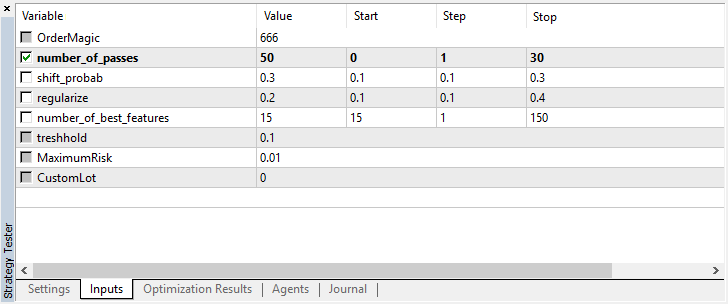Since the complexity of the calculations somewhat increased, I decided to stay with the following results after several iterations in the optimizer:

```2018.11.30 02:53:17.236 Core 2  2018.11.28 23:59:59   RlMonteCarloMulti TRAIN LOSS
2018.11.30 02:53:17.236 Core 2  2018.11.28 23:59:59   0.13229 0.16667 0.16262 0.14599 0.20937
2018.11.30 02:53:17.236 Core 2  2018.11.28 23:59:59   RlMonteCarloMulti OOB LOSS
2018.11.30 02:53:17.236 Core 2  2018.11.28 23:59:59   0.45377 0.45758 0.44650 0.45693 0.46120
```

The error on the test set remained quite considerable, however, at the threshold of 0.2, the model showed profit within 4 months, although it behaved rather unstable on the test data.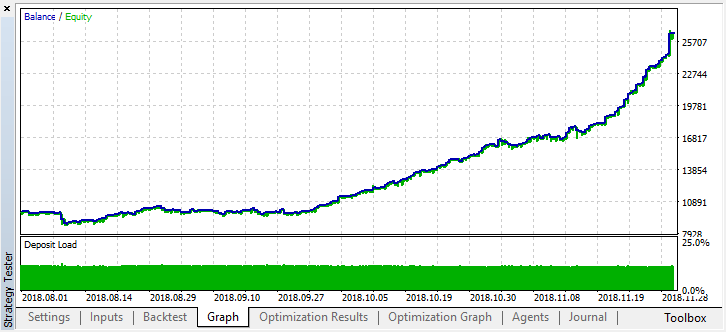Keep in mind that all learners were trained on the same data (Close prices), so there were no reasons to add new ones. Anyway, it was a simple example of adding new agents.

### Conclusions

Reinforcement learning is perhaps one of the most interesting methods of machine learning. It is always tempting to think that artificial intelligence is capable of solving trading problems in financial markets while learning independently. At the same time, one should have a broad knowledge of machine learning, statistics and probability theory in order to develop such a "miracle." The Monte Carlo method and selecting a model by the smallest error on test data have significantly improved the model offered in the first article. The model has become less overfitted.

The best model should be selected both in terms of the number of deals and the smallest classification error on the out-of-bag set. Ideally, errors in the training and test sets should be approximately equal and not reach the value of 0.5 (half of the examples are predicted incorrectly).

Translated from Russian by MetaQuotes Software Corp.
Original article: https://www.mql5.com/ru/articles/4777

Attached files |
RL_Monte_Carlo.mqh (17.26 KB)
Last comments | Go to discussion (15)| 30 Jul 2019 at 17:45
mastil55:

Hi Maxim, thank u for your work, i was trying to test your code, however it shows me some errors in the mq4 file with the following text

'getRDFstructure' - function already defined and has different type RL_Monte_Carlo.mqh 76 11

'RecursiveElimination' - function already defined and has different type RL_Monte_Carlo.mqh 133 11

'updatePolicy' - function already defined and has different type RL_Monte_Carlo.mqh 221 11

'updateReward' - function already defined and has different type RL_Monte_Carlo.mqh 236 11

'setAgentSettings' - function already defined and has different type RL_Monte_Carlo.mqh 361 12

'updatePolicies' - function already defined and has different type RL_Monte_Carlo.mqh 373 12

'updateRewards' - function already defined and has different type RL_Monte_Carlo.mqh 380 12

Do you know how to solve that issue?

hi, you just need to add "void" before all functions, just new compiler of MT5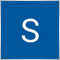| 25 Aug 2019 at 17:47

I am still trying to understand how to configure the inputs I am lay in the subject RL but it would be possible to apply this technique to the book of offers market depth as i done on NN sorry for newbie question!!! other thing is possible to use tensorflow model ! thanks in advance you are the best man!| 29 Aug 2019 at 22:16

Hi, I've been back-testing this code for hours. I wouldn't say it's learning much. The results seem pretty random with each pass and don't seem to progressively improve.

I would love to help out on this topic. I'm going to hack this code a bit and see what I can make it do. It's quite a learning curve for me at the moment but I find it intriguing.| 30 Aug 2019 at 01:32
chemmens:

Hi, I've been back-testing this code for hours. I wouldn't say it's learning much. The results seem pretty random with each pass and don't seem to progressively improve.

I would love to help out on this topic. I'm going to hack this code a bit and see what I can make it do. It's quite a learning curve for me at the moment but I find it intriguing.

I wish you good luck with hacking source code| 30 Aug 2019 at 01:35

I am still trying to understand how to configure the inputs I am lay in the subject RL but it would be possible to apply this technique to the book of offers market depth as i done on NN sorry for newbie question!!! other thing is possible to use tensorflow model ! thanks in advance you are the best man!

You can apply it to everything you want, it's just example with row price returns, without any exogenous info or order book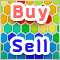Practical Use of Kohonen Neural Networks in Algorithmic Trading. Part II. Optimizing and forecasting

Based on universal tools designed for working with Kohonen networks, we construct the system of analyzing and selecting the optimal EA parameters and consider forecasting time series. In Part I, we corrected and improved the publicly available neural network classes, having added necessary algorithms. Now, it is time to apply them to practice.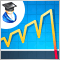Martingale as the basis for a long-term trading strategy

In this article we will consider in detail the martingale system. We will review whether this system can be applied in trading and how to use it in order to minimize risks. The main disadvantage of this simple system is the probability of losing the entire deposit. This fact must be taken into account, if you decide to trade using the martingale technique.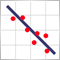Practical application of correlations in trading

In this article, we will analyze the concept of correlation between variables, as well as methods for the calculation of correlation coefficients and their practical use in trading. Correlation is a statistical relationship between two or more random variables (or quantities which can be considered random with some acceptable degree of accuracy). Changes in one ore more variables lead to systematic changes of other related variables.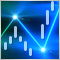The power of ZigZag (part I). Developing the base class of the indicator

Many researchers do not pay enough attention to determining the price behavior. At the same time, complex methods are used, which very often are simply “black boxes”, such as machine learning or neural networks. The most important question arising in that case is what data to submit for training a particular model.